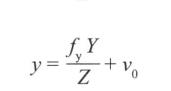# 聊一聊OpenCV相机标定

更新时间：2018年01月10日 11:22:01   作者：林多h0是物体的高，hi是图像上物体的高，f是焦距（距离），d0是图像到镜头的距离。四者满足如下关系：（1）（X，Y，Z）为点的三维坐标，（x，y）为其通过相机成像后在图像（二维）上的坐标。u0和v0是相机的中心点（主点)，该点位于图像平面中心（理论上是这样。但实际的相机会有几个像素的偏差）```std::vector<cv::Point2f>imageConers;
//提取标定图像角点，保存角点坐标（二维）
cv::findChessboardCorners(image,
boardSize, //角点数目如（6,4）六行，四列
imageConers);

```

```cv::calibrateCamera(objectPoints,//三维坐标
imagePoints, //二维坐标
imageSize,//图像大小
camerMatirx,//相机内参数矩阵
disCoeffs,//投影矩阵
rvecs, //旋转
tvecs,//平移
flag //标记opencv提供几种参数，可以参看在线的opencv document
);```

```//计算畸变参数
cv::initUndistortRectifyMap(camerMatirx, disCoeffs,
cv::Mat(), cv::Mat(), image.size(), CV_32FC1,
map1, //x映射函数
map2 //y映射函数
);
//应用映射函数
cv::remap(image, //畸变图像
undistorted, //去畸变图像
map1, map2, cv::INTER_LINEAR);

```

```#include<opencv2\core\core.hpp>
#include<opencv2\highgui\highgui.hpp>
#include<opencv2\imgproc\imgproc.hpp>
#include<opencv2\calib3d\calib3d.hpp>
#include <opencv2/features2d/features2d.hpp>
#include<string>
#include<vector>
class CameraCalibrator
{
private:
//世界坐标
std::vector < std::vector<cv::Point3f >> objectPoints;
//图像坐标
std::vector <std::vector<cv::Point2f>> imagePoints;
//输出矩阵
cv::Mat camerMatirx;
cv::Mat disCoeffs;
//标记
int flag;
//去畸变参数
cv::Mat map1, map2;
//是否去畸变
bool mustInitUndistort;

///保存点数据
{
imagePoints.push_back(imageConers);
objectPoints.push_back(objectConers);
}
public:
CameraCalibrator() :flag(0), mustInitUndistort(true){}
//打开棋盘图片，提取角点
{
std::vector<cv::Point2f>imageConers;
std::vector<cv::Point3f>objectConers;
//输入角点的世界坐标
for (int i = 0; i < boardSize.height; i++)
{
for (int j = 0; j < boardSize.width; j++)
{
objectConers.push_back(cv::Point3f(i, j, 0.0f));
}
}
//计算角点在图像中的坐标
cv::Mat image;
int success = 0;
for (int i = 0; i < filelist.size(); i++)
{
//找到角点坐标
bool found = cv::findChessboardCorners(image, boardSize, imageConers);
cv::cornerSubPix(image,
imageConers,
cv::Size(5, 5),
cv::Size(-1, -1),
cv::TermCriteria(cv::TermCriteria::MAX_ITER + cv::TermCriteria::EPS,
30, 0.1));
if (imageConers.size() == boardSize.area())
{
success++;
}
//画出角点
cv::drawChessboardCorners(image, boardSize, imageConers, found);
cv::imshow("Corners on Chessboard", image);
cv::waitKey(100);
}
return success;
}

//相机标定
double calibrate(cv::Size&imageSize)
{
mustInitUndistort = true;
std::vector<cv::Mat>rvecs, tvecs;
//相机标定
return cv::calibrateCamera(objectPoints, imagePoints, imageSize,
camerMatirx, disCoeffs, rvecs, tvecs, flag);
}
///去畸变
cv::Mat remap(const cv::Mat &image)
{
cv::Mat undistorted;
if (mustInitUndistort)
{
//计算畸变参数
cv::initUndistortRectifyMap(camerMatirx, disCoeffs,
cv::Mat(), cv::Mat(), image.size(), CV_32FC1, map1, map2);
mustInitUndistort = false;
}
//应用映射函数
cv::remap(image, undistorted, map1, map2, cv::INTER_LINEAR);
return undistorted;
}
//常成员函数，获得相机内参数矩阵、投影矩阵数据
cv::Mat getCameraMatrix() const { return camerMatirx; }
cv::Mat getDistCoeffs()  const { return disCoeffs; }
};
```

```#include"标头.h"
#include<iomanip>
#include<iostream>
int main()
{
CameraCalibrator Cc;
cv::Mat image;
std::vector<std::string> filelist;
cv::namedWindow("Image");
for (int i = 1; i <= 22; i++)
{
///读取图片
std::stringstream s;
s << "D:/images/chessboards/chessboard" << std::setw(2) << std::setfill('0') << i << ".jpg";
std::cout << s.str() << std::endl;

filelist.push_back(s.str());
cv::imshow("Image", image);
cv::waitKey(100);
}
//相机标定
cv::Size boardSize(6, 4);
Cc.calibrate(image.size());

//去畸变
cv::Mat uImage=Cc.remap(image);
cv::imshow("原图像", image);
cv::imshow("去畸变", uImage);
//显示相机内参数矩阵
cv::Mat cameraMatrix = Cc.getCameraMatrix();
std::cout << " Camera intrinsic: " << cameraMatrix.rows << "x" << cameraMatrix.cols << std::endl;
std::cout << cameraMatrix.at<double>(0, 0) << " " << cameraMatrix.at<double>(0, 1) << " " << cameraMatrix.at<double>(0, 2) << std::endl;
std::cout << cameraMatrix.at<double>(1, 0) << " " << cameraMatrix.at<double>(1, 1) << " " << cameraMatrix.at<double>(1, 2) << std::endl;
std::cout << cameraMatrix.at<double>(2, 0) << " " << cameraMatrix.at<double>(2, 1) << " " << cameraMatrix.at<double>(2, 2) << std::endl;

cv::waitKey(0);
}
```172.654 、0、157.829
0、184.195、118.635
0 、0 、1